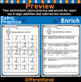Subject
Resource Type
File Type

PDF

(7 MB|20 pages)
Product Rating
4.0
(4 Ratings)
Standards
Also included in:
1. Great extra practice pages for your first grade classroom! This is a FULL YEAR of math worksheets to go with your math curriculum. This includes 12 units of study (see below). These worksheet lessons are a great resource for extra practice! If you need to differentiate math centers, or challenge
\$36.50
\$30.00
Save \$6.50
• Product Description
• StandardsNEW

2-digit addition and subtraction practice! These worksheet lessons are a great resources for extra practice with learning strategies for two-digit addition and subtraction! If you need to differentiate math centers, or challenge students these worksheets provide an enrich page for each lesson. Great resource for math centers, morning work, or homework. These worksheets are meant to complement your lessons. Use them for further instruction or practice with each concept. These worksheets are a go to when practicing the math skills listed below!

Lessons included in worksheets:

1. Add and Subtract within 20

3. Subtract Tens

4. Use a Hundreds Chart to Add

6. Make a Ten to Add

7. Use Place Value to Add

10. Practice 2-digit Addition and Subtraction

This download includes 20 worksheets based on addition and subtraction with 2-digit numbers. There are 2 worksheets for each lesson listed above. The 2 worksheets include one for extra Practice (on track) and one for Enrich (challenging).

***Try my other first grade math worksheets***

Subtraction Strategies Worksheets

Count and Model Numbers Worksheets

Comparing Numbers - Greater Than, Less Than, Equal to

Subtract multiples of 10 in the range 10-90 from multiples of 10 in the range 10-90 (positive or zero differences), using concrete models or drawings and strategies based on place value, properties of operations, and/or the relationship between addition and subtraction; relate the strategy to a written method and explain the reasoning used.
Add within 100, including adding a two-digit number and a one-digit number, and adding a two-digit number and a multiple of 10, using concrete models or drawings and strategies based on place value, properties of operations, and/or the relationship between addition and subtraction; relate the strategy to a written method and explain the reasoning used. Understand that in adding two-digit numbers, one adds tens and tens, ones and ones; and sometimes it is necessary to compose a ten.
10 can be thought of as a bundle of ten ones - called a “ten.”
Understand that the two digits of a two-digit number represent amounts of tens and ones. Understand the following as special cases:
Count to 120, starting at any number less than 120. In this range, read and write numerals and represent a number of objects with a written numeral.
Total Pages
20 pages
N/A
Teaching Duration
N/A
Report this Resource to TpT
Reported resources will be reviewed by our team. Report this resource to let us know if this resource violates TpT’s content guidelines.